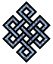#fail2ban bad ip database: ip 35.229.22.38

### | ip database | live view | stats | report | help | api key:

 ip: 35.229.22.38 hostname: 38.22.229.35.bc.googleusercontent.com country:[US] United States first reported: 21.11.2018 00:38.20 GMT+0200 last reported: 24.11.2018 02:21.51 GMT+0200 time period: 3d 01h 43m 31s total reports: 10 reported by: 1 host(s) filter(s): ssh (10) tor exit node no badips.com db Lookup## port scan of '35.229.22.38':

[-hide]
```# Nmap 6.40 scan initiated Wed Nov 21 00:39:02 2018 as: /usr/bin/nmap -sU -sS -O 35.229.22.38
Nmap scan report for 38.22.229.35.bc.googleusercontent.com (35.229.22.38)
Host is up (0.092s latency).
Not shown: 1000 open|filtered ports, 993 filtered ports
PORT     STATE  SERVICE
21/tcp   closed ftp
22/tcp   open   ssh
80/tcp   open   http
443/tcp  closed https
3389/tcp closed ms-wbt-server
8000/tcp closed http-alt
8001/tcp closed vcom-tunnel
No exact OS matches for host (If you know what OS is running on it, see http://nmap.org/submit/ ).
TCP/IP fingerprint:
OS:SCAN(V=6.40%E=4%D=11/21%OT=22%CT=21%CU=%PV=N%G=Y%TM=5BF49B7D%P=x86_64-pc
OS:-linux-gnu)SEQ(SP=FF%GCD=1%ISR=10C%TI=Z%CI=I%TS=8)SEQ(SP=FF%GCD=2%ISR=10
OS:C%TI=Z%TS=8)OPS(O1=M58CST11NW7%O2=M58CST11NW7%O3=M58CNNT11NW7%O4=M58CST1
OS:1NW7%O5=M58CST11NW7%O6=M58CST11)WIN(W1=6E00%W2=6E00%W3=6E00%W4=6E00%W5=6
OS:E00%W6=6E00)ECN(R=Y%DF=Y%TG=40%W=6EF0%O=M58CNNSNW7%CC=Y%Q=)T1(R=Y%DF=Y%T
OS:G=40%S=O%A=S+%F=AS%RD=0%Q=)T2(R=N)T3(R=N)T4(R=Y%DF=Y%TG=40%W=0%S=A%A=Z%F
OS:=R%O=%RD=0%Q=)T5(R=Y%DF=Y%TG=40%W=0%S=Z%A=S+%F=AR%O=%RD=0%Q=)T6(R=Y%DF=Y
OS:%TG=40%W=0%S=A%A=Z%F=R%O=%RD=0%Q=)T7(R=Y%DF=Y%TG=40%W=0%S=Z%A=S+%F=AR%O=
OS:%RD=0%Q=)U1(R=N)IE(R=Y%DFI=N%TG=40%CD=S)

OS detection performed. Please report any incorrect results at http://nmap.org/submit/ .
# Nmap done at Wed Nov 21 00:40:45 2018 -- 1 IP address (1 host up) scanned in 104.00 seconds
```
```Σ = 34 | Δt = 0.004133939743042s
```